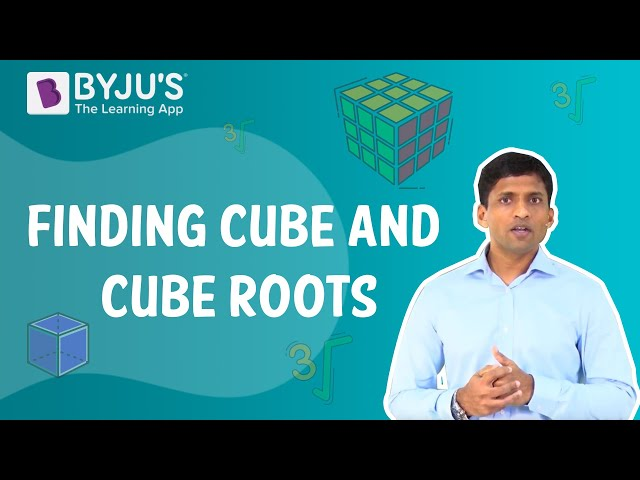Checkout JEE MAINS 2022 Question Paper Analysis : Checkout JEE MAINS 2022 Question Paper Analysis :

# Cube Root of 1728

The cube root of 1728, expressed as 3√1728, is equal to a value which when multiplied three times by itself will give the original number. To find the value of 3√1728, we can use two methods, i.e. prime factorisation method and estimation method, without using any calculator. Also, learn cube root of numbers here.

 Cube root of 1728, 3√1728 = 12

Since 1728 is a four-digit number, so finding its prime factors can be a little lengthy. Thus, we can use an estimation method for fast calculation. For this method, we have to learn the value of cubes of natural numbers from 1 to 10, which is provided here in the later part. Now, let us look at both the methods one by one.

Also, check:

## Cube Root of 1728 by Prime Factorisation Method

Using prime factorisation, we will find prime factors of 1728, since it is a perfect cube and then will pair them in a group of three. Let us understand it in a step by step procedure.

Step 1: Find the prime factors of 1728

1728 = 2x2x2x2x2x2x3x3x3

Step 2: Group the factors in a pair of three and write in the form of cubes.

1728 = (2x2x2)x(2x2x2)x(3x3x3)

1728 = 23x23x33

Step 3: Apply cube root both the sides and take out the terms in cubes out of the cube root.

3√1728 = 3√(23x23x33) = 2 x 2 x 3 = 12

Hence, 3√1728 =12

## Cube Root of 1728 By Estimation Method

Now, we will learn here to find the cube root of four-digit number 1728 using estimation method. Before we get to know about this method, we need to memorise the value of cubes from 1 to 10. Here is the table for reference.

 Number Cubes 1 1 2 8 3 27 4 64 5 125 6 216 7 343 8 512 9 729 10 1000

Now we will proceed to find the value of 3√1728 following these steps:

Step 1:

We will firstly take the digit at the unit’s place.

So, here we get 8.

Step 2:

Now we will check the cubes table, the cube of which number has 8 at its unit digit place.

We see, 23 = 8.

That means the cube root of 1728 will have 2 at the unit place.

Step 3:

Now ignore the last 3 digits of 1728, i.e. 728

Taking 1 as a reference number, we know the cube of 1 is equal to 1.

Therefore, we get the two-digit of the answer.

Hence, 3√1728 = 12

## Video Lesson on Finding Cube Roots